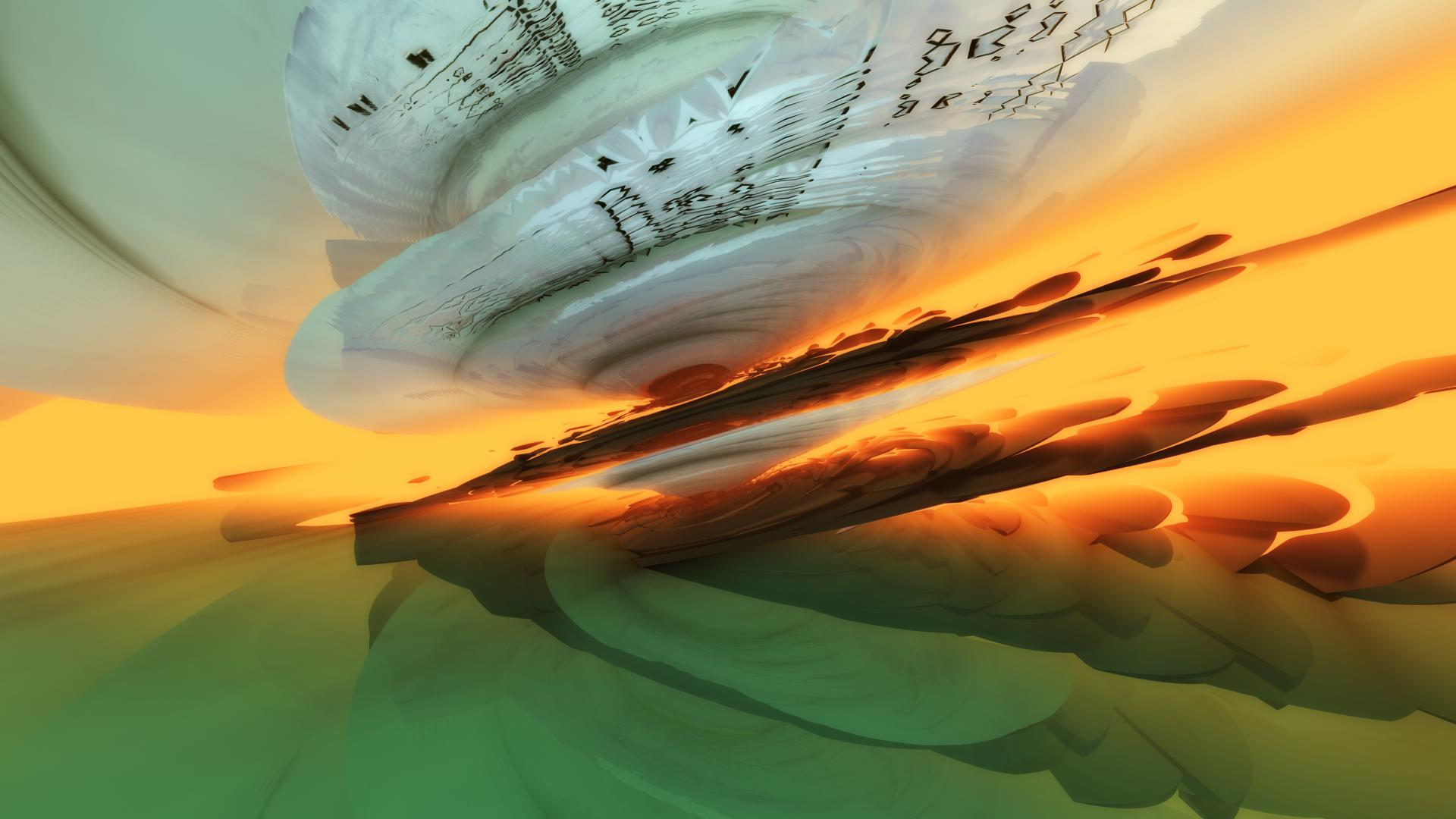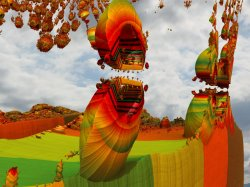News: Did you know ? you can use LaTex inside Postings on fractalforums.com!## The All New FractalForums is now in Public Beta Testing! Visit FractalForums.org and check it out!

Landing at NastapokaPrevious Image | Next Image
Description: From Crist-JRogers PKM03 frag

FOV = 1.00588
Eye = -2.22783,-5.81824,2.37611
Target = -2.96063,0.0373558,0.665701
FocalPlane = 1
Aperture = 0
InFocusAWidth = 0
ApertureNbrSides = 7
ApertureRot = 0
ApStarShaped = false
Gamma = 1.35745
ToneMapping = 5
Exposure = 0.93918
Brightness = 0.8194
Contrast = 1.54465
Saturation = 2.35945
GaussianWeight = 1
AntiAliasScale = 2
Bloom = true
BloomIntensity = 0.5536
BloomPow = 3.3693
BloomTaps = 20
Detail = -4.12055
DetailAO = -0.4508
FudgeFactor = 0.53189
MaxRaySteps = 2000
MaxDistance = 151.55
Dither = 0.89841
NormalBackStep = 1
AO = 0,0,0,1
AoCorrect = 0
Specular = 0
SpecularExp = 16
CamLight = 0.772549,0.894118,1,0.67648
CamLightMin = 1
Glow = 0.905882,1,0.823529,0
GlowMax = 20
Reflection = 0.352941,0.403922,0.313725
ReflectionsNumber = 4
SpotGlow = true
SpotLight = 1,0.847059,0.952941,5.3382
LightPos = 15,3.9326,-7.6674
LightSize = 1
LightFallOff = 0.04796
LightGlowExp = 1.76835
BaseColor = 1,1,1
OrbitStrength = 0.2349
X = 0.411765,0.6,0.556863,0.75268
Y = 0.592157,0.666667,0.592157,0.87634
Z = 0.937255,0.905882,1,0.64566
R = 0.666667,0.666667,0.498039,0.931
BackgroundColor = 0.482353,0.682353,0.831373
CycleColors = true
Cycles = 2.62255
EnableFloor = true
FloorNormal = -1,-0.1282,0.21494
FloorHeight = 1.3981
FloorColor = 0.407843,0.541176,0.439216
HF_Fallof = 1.39191
HF_Const = 0.03522
HF_Intensity = 0.37108
HF_Dir = -0.06642,-1,-0.06168
HF_Offset = 0.8904
HF_Color = 0.686275,0.498039,0.345098,1.38714
HF_Scatter = 10
HF_Anisotropy = 0,0,0
HF_FogIter = 4
CloudScale = 1.31879
CloudFlatness = 0
CloudTops = 10
CloudBase = 2.1518
CloudDensity = 0.52632
CloudRoughness = 1
CloudContrast = 5.9375
CloudColor = 0.65,0.68,0.7
SunLightColor = 0.7,0.5,0.3
MI = 38
MI2 = 50
v1 = 0.7706
v2 = 3.9834,2.128,1.4049
v3 = 0.73243
w1 = 1.03134,0.81638,0.79952
Size = 0.1267
CSize = 0.11436,0,0.0075
C = 1.16288,-0.04488,-0.08736
DEoffset = 0.00027
Offset = 0,0,0
ColorIterations = 5
iIterations = 7
iScale = 1.50892
Bailout = 177.488
iSize = 1.64284
plnormal = 0.84226,-0.99518,-0.06924
iOffset = 0.2506,0.15132,0
Angle1 = 55.2492
Rot1 = 0.86054,0.99046,0.99642
Angle2 = 11.304
Rot2 = 0.80572,1,1
Up = -1.38398,0.914027,0.0795166
Stats:
Total Favorities: 0 View Who Favorited
Filesize: 140.43kB
Height: 1080 Width: 1920
Discussion Topic: View Topic
Keywords: timemit fragmentarium pseudo kleinian menger spacecraft crash crater
Posted by: Tim EmitJanuary 14, 2016, 04:42:34 PM

Rating: Has not been rated yet.

 The LandingRating:Filesize: 494.51kBDate: January 27, 2011, 01:32:31 PMComments (0)By: Power 8 Arctic night, Landing in a Winter wonderland.Rating:Filesize: 420kBDate: December 05, 2015, 09:59:32 AMComments (0)By: Caleidoscope Lunacy LandingRating:Filesize: 234.69kBDate: February 03, 2016, 09:30:46 PMComments (1)By: scratchytag Lumpkins Landing In La La Land IIRating:Filesize: 417.7kBDate: August 22, 2016, 02:59:39 AMComments (0)By: mclarekin